Date: Sun, 11 Jan 1998 11:25:32 -0600 (CST)

Name: james

Who is asking: Student
Level: Secondary

Question:

If n is a positive integer such that 2n has 28 positive divisors and 3n has 30 positive divisors, then how many positive divisors does 6n have?
(a) 32 (b) 34 (c) 35 (d) 36 (e) 38

Hi James,

We have two solutions for you. One by Penny and a second by Haragauri.

Penny's Solution:

If you write n as a product of powers of primes, i.e.,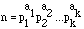then a divisor m of n must be of the form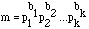where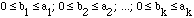Thus, if you write d(n) for the number of divisors of n then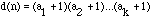Writing a^b for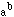and * for multiplication we see for example that if n = 2^4*5^7*11^2 then d(n) = 5*8*3 = 120.

Since d(2n) = 28 and 28 can be written as a product of integers larger than 1 in four ways, (2x2x7, 2x14, 4x7, and 28), we get the following table of possibilities. (Notice that since 2 divides 2n, 2 is one of the prime factors of 2n.)

d(2n)=282nn
2x2x72*p*q^6p*q^6
p*q*2^6p*q*2^5
2x142*p^13p^13
p*2^13p*2^12
4x72^3*p^62^2*p^6
p^3*2^6p^3*2^5
282^272^26
In this table p and q are distinct primes neither of which is 2.

Applying the same reasoning to d(3n)=30 gives

d(3n)=303nn
2x3x53*s^2*t^4s^2*t^4
3^2*s*t^43*s*t^4
s*t^2*3^43^3*s*t^2
6x53^5*s^43^4*s^4
3^4*s^53^3*s^5
3x103^2*s^93*s^9
s^2*3^9s^2*3^8
2x153*s^14s^14
3^14 s3^13 s
303^293^28
In this table s and t are distinct primes neither of which is 3.

Examination of the options for n in the two tables shows that the only possibility is n=2^5 3^3 and thus 6n=2^6 3^4 and hence d(6n)=7x5=35.

Haragauri's Solution:

Suppose n=(2^s)*(3^t)*Q where Q is an integer not divisible by 2 or 3 then, using the the function d defined in Penny's solution, d(n) = d(2^s)*d(3^t)*d(Q). Hence d(2*n)=d(2^(s+1))*d(3^t)*d(q) = 28 or
(s+2)(t+1)d(Q)=28, and
d(3*n)=d(2^s)*d(3^(t+1))*d(q) = 30 or
(s+1)(t+2)d(Q)=30.
Thus d(Q) divides both 28 and 30 and hence d(Q) divides 2=gcd(28,30). Hence d(Q) is either 1 or 2.

Suppose d(Q)=2 then
(s+2)(t+1)=14 and
(s+1)(t+2)=15. Subtracting these equation yields
s-t=1 or s=t+1. Thus
14=(s+2)(t+1)=(s+2)s which is impossible since s is an integer. Thus d(Q)=1 and hence Q=1.

Since d(Q)=1,
(s+2)(t+1)=28 and
(s+1)(t+2)=30. Again subrtacting gives
s-t=2 or s=t+2. Thus
30=(s+1)(t+2)=(s+1)s and therefore s=5 and t=3. hence, as in Penny's solution
n = (2^5)(3^3)

Cheers.

Go to Math Central

To return to the previous page use your browser's back button.